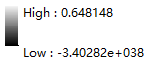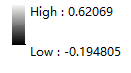# Saving rasters which are extracted by mask using python will change the results and generate some abnormal values

914
4
10-12-2019 10:34 AMby
New Contributor II

Hi,

I am trying to extract the rasters by mask in batches using Python in ArcGIS 10.3. When I extracted one sample raster data by mask using the Extract by Mask tool in ArcToolbox, the result is what I expected. But I have too much raster data to extracted one by one, so I used this code to achieve it:

``````import arcpy
from arcpy import env
from arcpy.sa import *

#environment settings
env.workspace = "E:/MGH_data/MGH_newLandsat/Bulk Order 1043142/NDVI_TIFF"
rasterList = arcpy.ListRasters("*","tif")

#out put path
output_path = "E:/MGH_data/MGH_newLandsat/Bulk Order 1043142/NDVI_Clip/"

for raster in rasterList:
print raster
inRaster = raster
# Save the output
outname = output_path + inRaster
‍‍‍‍‍‍‍‍‍‍‍‍‍‍‍‍‍‍‍‍‍‍‍‍‍‍‍‍‍‍‍‍‍‍‍‍‍‍‍‍‍‍‍‍‍‍‍‍‍‍‍‍‍‍‍‍‍‍‍‍‍‍‍‍‍‍‍‍‍‍‍‍‍‍‍‍‍‍‍‍‍‍‍‍‍‍‍‍‍‍‍‍‍‍‍‍‍‍‍‍‍‍‍‍‍‍‍‍‍‍‍‍‍‍‍‍‍‍‍‍‍‍‍‍‍‍‍``````

when I execute the above commands in Python window,  there are some abnormal values in the nodata area in the results. Here is the value range of the result with abnormal values (the low value)So I was wondering which step is wrong. So I tried to use this code to extract one sample raster data, and I just ran the code only before saving the output, which means it will not to execute the code that is used to save the output (the last two lines of the code), and the result is correct, without any abnormal values. Here is the value range of the correct raster result:So I guess the problem is from the Save output raster step. But I checked the code many times and still have no idea to solve it. Anybody knows what's going wrong? I do appreciate your help.

Thanks!

Tags (2)
1 Solution

Accepted Solutionsby
New Contributor II

Hi, Dan. I tried an another method and it finally worked.

I ran the code using Python in ArcGIS 10.3 before. But this time, I saved my code as a Python File (*.py) and ran it using the IDLE, and finnaly I got the results which are what I want. And the code I used this time is a little different from that I post above. Here is the code I used this time:

``````import arcpy
import glob
import os

arcpy.CheckOutExtension('Spatial')

from arcpy import env
from arcpy.sa import *

env.workspace = r"C:/Users/xuli9/Desktop/NDVI/NDVI_TIFF"
inws = r"C:/Users/xuli9/Desktop/NDVI/NDVI_TIFF"
rasterList = glob.glob(os.path.join(inws, "*.tif"))

output_path = r"C:/Users/xuli9/Desktop/NDVI/NDVI_Extract/"

for raster in rasterList:
print raster
inRaster = raster
arcpy.env.snapraster = inRaster
outname = os.path.join(output_path, os.path.basename(inRaster).split(".") + "_clp.tif")
out_Extract.save(outname)
‍‍‍‍‍‍‍‍‍‍‍‍‍‍‍‍‍‍‍‍‍‍‍``````

I post my solution and hope it could help someone who may meet this kind of problems.

Thank you again, Dan!

4 RepliesbyMVP Esteemed Contributor

I would convert the shapefile to a raster and ensure that you specify the exact same cell size and snap raster to ensure that the raster cells align and avoid internal resamplingby
New Contributor II

Thanks Dan.

As you say, I used the raster as the mask to extract my raster data and also specified the snap raster. But it still doesn't work and still exist some abnormal values in the nodata area in the result. Here is the code:

``````import arcpy
from arcpy import env
from arcpy.sa import *
env.workspace = "E:/MGH_data/MGH_newLandsat/Bulk Order 1043142/NDVI_TIFF"
rasterList = arcpy.ListRasters("*","tif")

output_path = "E:/MGH_data/MGH_newLandsat/Bulk Order 1043142/NDVI_Clip/"
#use the raster as the mask

for raster in rasterList:
print raster
inRaster = raster
arcpy.env.snapraster = inRaster
outname = output_path + inRaster

I really think the problem is from the raster save step. I'd appreciate it if you have some other suggestions to solve this problem.

Thanks!by
New Contributor II

Hi, Dan. I tried an another method and it finally worked.

I ran the code using Python in ArcGIS 10.3 before. But this time, I saved my code as a Python File (*.py) and ran it using the IDLE, and finnaly I got the results which are what I want. And the code I used this time is a little different from that I post above. Here is the code I used this time:

``````import arcpy
import glob
import os

arcpy.CheckOutExtension('Spatial')

from arcpy import env
from arcpy.sa import *

env.workspace = r"C:/Users/xuli9/Desktop/NDVI/NDVI_TIFF"
inws = r"C:/Users/xuli9/Desktop/NDVI/NDVI_TIFF"
rasterList = glob.glob(os.path.join(inws, "*.tif"))

output_path = r"C:/Users/xuli9/Desktop/NDVI/NDVI_Extract/"

for raster in rasterList:
print raster
inRaster = raster
arcpy.env.snapraster = inRaster
outname = os.path.join(output_path, os.path.basename(inRaster).split(".") + "_clp.tif")
out_Extract.save(outname)
‍‍‍‍‍‍‍‍‍‍‍‍‍‍‍‍‍‍‍‍‍‍‍``````

I post my solution and hope it could help someone who may meet this kind of problems.

Thank you again, Dan!byMVP Esteemed Contributor

That is the route to take Li.  Thanks for following up